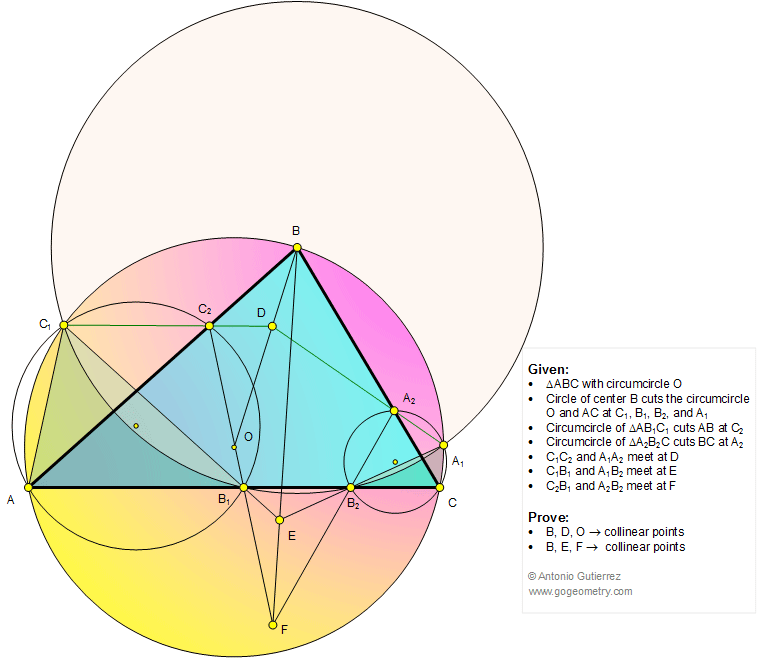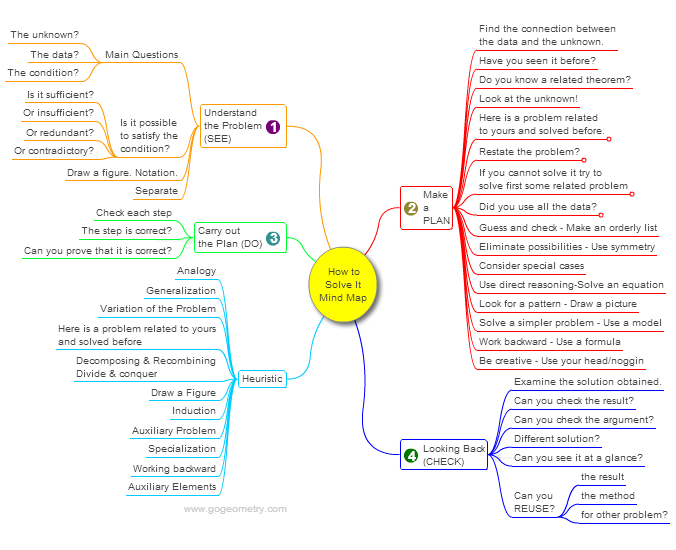# Geometry Problem 1140: Triangle, Circumcircle, Circle, Center, Collinear Points. Level: High School, SAT Prep, Honors Geometry, College, Math Education

< PREVIOUS PROBLEM  |  NEXT PROBLEM >

In a triangle ABC of circumcircle O, a circle of center B cuts the circle O and AC at C1, B1, B2, and A1, respectively, (see figure below). The circumcircle of triangle AB1C1 cuts AB at C2. The circumcircle of triangle A2B2C cuts BC at A2. C1C2 and A1A2 meet at D. C1B1 and A1B2 meet at E. C2B1 and A2B2 meet at F. Prove that (1) Points B, D, and O are collinear; (2) Points B, E, and F are collinear.## Mind Map: George Pólya's 1945 book, "How to Solve It"

George Pólya's 1945 book "How to Solve It, A new aspect of Mathematical Method", is a book describing methods of problem solving. It suggests the following steps when solving a mathematical problem: (1) First, you have to understand the problem. (2) After understanding, then make a plan. (3) Carry out the plan. (4) Look back on your work. How could it be better?
A mind map is a diagram used to visually organize information, knowledge, concepts or ideas.Home | Search | Geometry | Problems | All Problems | Open Problems | Visual Index | 10 Problems | 1131-1140 | Triangle | Circle | Circumcircle | Collinear points | Email | Solution / comment.Last updated: Jul 18, 2015 by Antonio Gutierrez.